Definitions

# Spatial Geometry

Spatial geometry is the analysis of solids in space , that is, it is geometry for three-dimensional objects, as opposed to plane geometry, which is the study of two-dimensional figures Like the latter, the former arises based on primitive concepts, namely: point, line, plane and space.

Based on the primitive elements, geometric solids are developed, the main ones being polyhedrons : parallelepipeds, cubes and other prisms, in addition to those known as Plato’s solids; and round bodies : cone, cylinder and sphere. In addition to recognizing these solids, it is important to understand that volume and total area calculations have specific formulas for each type.

## Features of Spatial Geometry

Spatial Geometry studies objects that have more than one dimension and occupy space. In turn, these objects are known as ” geometric solids ” or ” spatial geometric figures “. Get to know some of them better:

• prism
• cube
• paving stone
• pyramid
• cone
• cylinder
• ball

In this way, spatial geometry is able to determine, through mathematical calculations, the volume of these same objects, that is, the space occupied by them.

However, the study of the structures of spatial figures and their interrelations is determined by some basic concepts , namely:

• Point : fundamental concept to all subsequent ones, since they are all, ultimately, formed by countless points. In turn, the points are infinite and have no measurable dimension (dimensionless). Therefore, its only guaranteed property is its location.
• Line : composed of points, it is infinite on both sides and determines the shortest distance between two given points.
• Line : has some similarities with a straight line, as it is equally infinite on each side, however, it has the property of forming curves and knots on itself.
• Flat : is another infinite structure that extends in all directions.

## Spatial Geometric Figures

Below are some of the most well-known spatial geometric figures:

### Cube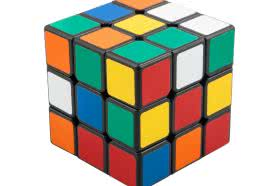The cube is a regular hexahedron composed of 6 square faces, 12 edges and 8 vertices:

Lateral area : 4a 2
Total area : 6a 2
Volume : aaa = a 3

### Dodecahedron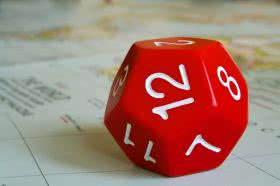The Dodecahedron is a regular polyhedron composed of 12 pentagonal faces, 30 edges and 20 vertices:

Total Area : 3√25+10√5a 2
Volume : 1/4 (15+7√5) to 3

### Tetrahedron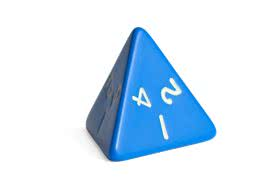The Tetrahedron is a regular polyhedron composed of 4 triangular faces, 6 edges and 4 vertices:

Total area : 4a 2 √3/4
Volume : 1/3 Ab.h

### OctahedronThe Octahedron is a regular polyhedron of 8 faces formed by equilateral triangles, 12 edges and 6 vertices being:

Total area : 2a 2 √3
Volume : 1/3 to 3 √2

### icosahedron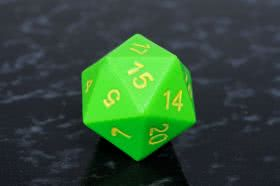The Icosahedron is a convex polyhedron composed of 20 triangular faces, 30 edges and 12 vertices:

Total area : 5√3a 2
Volume : 5/12 (3+√5) a 3

### Prism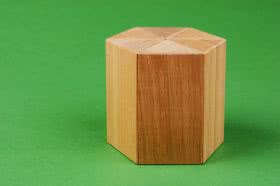The Prism is a polyhedron composed of two parallel faces that form the base, which in turn can be triangular, quadrangular, pentagonal, hexagonal.

In addition to the faces, the prima is composed of height, sides, vertices and edges joined by parallelograms. According to their inclination, the prisms can be straight, those in which the edge and the base make an angle of 90º or the oblique ones composed of angles different from 90º.

Face Area : ah
Side Area : 6.ah
Base Area : 3.a 3 √3/2
Volume : Ab.h

Where:
Ab : Area of ​​the base
h : Height

### PyramidThe pyramid is a polyhedron composed of a base (triangular, pentagonal, square, rectangular, parallelogram), a vertex (vertex of the pyramid) that unites all the lateral triangular faces.

Its height corresponds to the distance between the vertex and its base. As for their inclination, they can be classified as straight (angles of 90º) or oblique (angles other than 90º).

Total area : Al + Ab
Volume : 1/3 Ab.h

Where:

Al : Lateral area
Ab : Base area
h : height

## Curiosities

• The word “geometry” comes from the Greek and corresponds to the union of the terms “geo” of earth and “metria” of measure, which means “to measure earth.”
• The most common calculations in spatial geometry are to determine the lengths of curves, areas of surfaces and volumes of solid regions.
• Other spatial geometric figures: cylinder, cone, sphere.
• The “Platonic Solids” are convex polyhedra known since classical antiquity. The five “Platonic solids” are: tetrahedron, cube, octahedron, dodecahedron, icosahedron.

Check Also
Close